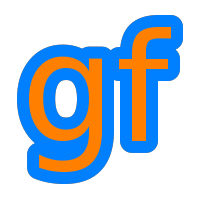Gamedev Framework (gf)  0.4.0 A C++11 framework for 2D games
gf::Circ< T > Struct Template Reference

Utility class for manipulating circles. More...

#include <gf/Circ.h>

## Public Member Functions

constexpr Circ () noexcept
Default constructor. More...

constexpr Circ (const Vector< T, 2 > &circCenter, T circRadius) noexcept
Construct the circle from center and radius. More...

constexpr Vector< T, 2 > getCenter () const noexcept
Get the center of the circle. More...

constexpr T getRadius () const noexcept
Get the radius of the circle. More...

constexpr bool isEmpty () const noexcept
Check if the circle is empty. More...

constexpr Vector< T, 2 > getTop () const noexcept
Get the top of the circle. More...

constexpr Vector< T, 2 > getBottom () const noexcept
Get the bottom of the circle. More...

constexpr Vector< T, 2 > getLeft () const noexcept
Get the left of the circle. More...

constexpr Vector< T, 2 > getRight () const noexcept
Get the right of the circle. More...

bool contains (const Vector< T, 2 > &point) const noexcept
Check if a point is insied a circle's area. More...

bool intersects (const Circ< T > &other) const noexcept
Check the intersection between two circles. More...

## Public Attributes

Vector< T, 2 > center
Center of the circle. More...

## Related Functions

(Note that these are not member functions.)

template<typename T >
bool operator== (const Circ< T > &lhs, const Circ< T > &rhs)
Equality operator. More...

template<typename T >
bool operator!= (const Circ< T > &lhs, const Circ< T > &rhs)
Inequality operator. More...

## Detailed Description

### template<typename T> struct gf::Circ< T >

Utility class for manipulating circles.

A circle is defined by its center and its radius. It is a very simple class defined for convenience, so its member variables (center and radius) are public and can be accessed directly.

gf::Circ is a template and may be used with any numeric type, but for simplicity, some common typedef are defined:

• gf::CircI with int as T
• gf::CircU with unsigned as T
• gf::CircZ with std::size_t as T
• gf::CircF with float as T

So that you don't have to care about the temlate syntax.

Usage example:

// Define a circle, with a center at (10, 10) and a radius of 20
gf::CircI c1({ 10, 10 }, 20);
// Define a circle with a center at (0, 0) and a radius of 2
gf::CircI c2({ 0, 0 }, 2);
// Test intersections with the point (3, 1)
bool b1 = c1.contains({ 3, 1 }); // true
bool b2 = c2.contains({ 3, 1 }); // false
// Test the intersection between c1 and c2
bool b3 = c1.intersects(c2); // true

## Constructor & Destructor Documentation

template<typename T>
 constexpr gf::Circ< T >::Circ ( )
inlinenoexcept

Default constructor.

Creates an empty circle (it is equivalent to calling Circ({ 0, 0 }, 0).

template<typename T>
 constexpr gf::Circ< T >::Circ ( const Vector< T, 2 > & circCenter, T circRadius )
inlinenoexcept

Construct the circle from center and radius.

Parameters

## Member Function Documentation

template<typename T>
 bool gf::Circ< T >::contains ( const Vector< T, 2 > & point ) const
inlinenoexcept

Check if a point is insied a circle's area.

Parameters
 point Point to test
Returns
True if the point is inside, false otherwise
intersects()
template<typename T>
 constexpr Vector gf::Circ< T >::getBottom ( ) const
inlinenoexcept

Get the bottom of the circle.

Returns
The bottom of the circle
template<typename T>
 constexpr Vector gf::Circ< T >::getCenter ( ) const
inlinenoexcept

Get the center of the circle.

It is a synonym for the center member

Returns
The center of the circle
template<typename T>
 constexpr Vector gf::Circ< T >::getLeft ( ) const
inlinenoexcept

Get the left of the circle.

Returns
The left of the circle
template<typename T>
 constexpr T gf::Circ< T >::getRadius ( ) const
inlinenoexcept

Get the radius of the circle.

It is a synonym for the radius member

Returns
getCenter()
template<typename T>
 constexpr Vector gf::Circ< T >::getRight ( ) const
inlinenoexcept

Get the right of the circle.

Returns
The right of the circle
template<typename T>
 constexpr Vector gf::Circ< T >::getTop ( ) const
inlinenoexcept

Get the top of the circle.

Returns
The top of the circle
template<typename T>
 bool gf::Circ< T >::intersects ( const Circ< T > & other ) const
inlinenoexcept

Check the intersection between two circles.

Parameters
 other Circle to test
Returns
True if circles overlap, false otherwise
contains()
template<typename T>
 constexpr bool gf::Circ< T >::isEmpty ( ) const
inlinenoexcept

Check if the circle is empty.

An empty circle is a circle with a zero radius.

Returns
True if the circle is empty

## Friends And Related Function Documentation

template<typename T >
 bool operator!= ( const Circ< T > & lhs, const Circ< T > & rhs )
related

Inequality operator.

Parameters
 lhs First circle rhs Second circle
Returns
True if the two circles are different
template<typename T >
 bool operator== ( const Circ< T > & lhs, const Circ< T > & rhs )
related

Equality operator.

Parameters
 lhs First circle rhs Second circle
Returns
True if the two circles are the same

## Member Data Documentation

template<typename T>
 Vector gf::Circ< T >::center

Center of the circle.

template<typename T>